|

# 颜少清:将中式建筑设计标准化,有利于更大范围的推广

我对时下流行的中式设计不太喜欢，可能是由于媒体总把中式设计与诸如文化复兴的重大责任关联甚至等同起来，过于沉重，也过于牵强。在我看来，喜好大红大紫还是喜好自然写意，喧嚣也好肤浅也罢，这就是当下的状态，如果设计要表达和传达什么，那也应该是把当代人的这种状态如实或者矫饰之后呈现出来，文化意义就由后世去评定吧！

当然，我是小辈，观点可能有失偏颇，所以我去看了一些中式设计做得好的、以及在设计中的中式痕迹不那么明显的前辈设计师的相关言论，比如梁志天老师，梁建国老师和琚宾老师。梁志天老师认为本土化很好，国际化也很好；被认为中式设计做得很极致的梁建国老师认为无关中西，设计即生活，设计师先要对生活有真正的理解，否则做出来的设计就会是肤浅而表面的；琚宾老师也是认为设计首要的任务是解决问题，然后才考虑艺术和文化因素。最让我有共鸣的是潘向东老师的观点，“有些人坚持东方的设计，值得尊敬，但也不需要每一个设计师背着沉重的文化任务，顺其自然就好。”

带着对中式设计的一点理解和更多的疑问，我采访了颜少清设计师，他是上海泽钦室内设计有限公司合伙人及设计师，很有传统设计师的文化和社会责任感，“致力于作为中国文化传统文化的传承者，感悟并睿智——通过这种状态获得了一个极佳的角度来描绘本土新兴生活。”他主持的项目包括中国院子、合肥院子、姑苏院子、福州金尊府、融创使馆一号院等。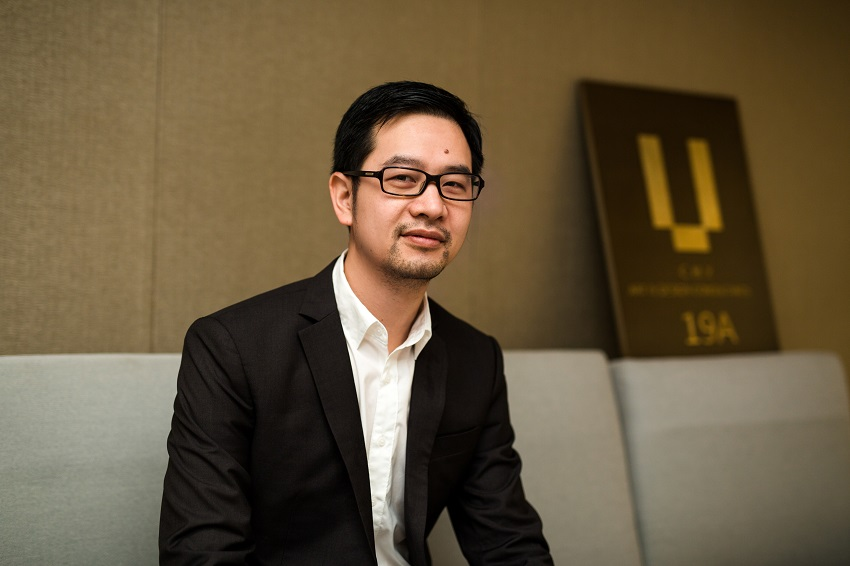上海泽钦室内设计有限公司合伙人及设计师颜少清

在和设计师的沟通中，我修订了一些对中式设计的理解，不必过于吹捧或者担忧，每个业主对中式设计的理解不同，一块砖、一片瓦，他要留住和呈现的可能就是他记忆里一点久远的感动；每个设计师对中式设计的理解也不同，深刻或肤浅，也难免片面，当下即是明日的历史，以下为采访实录。

【搜狐焦点家居】：主要的设计理念是什么？

【颜少清】：在设计中我们整体考虑，审视历史、现在和未来，做一些实验性的突破，希望在设计中做出传统的空间，去承载我们的历史，而在局部做的一些突破，则希望能够达到量变到质变的效果。

【搜狐焦点家居】：当下新中式流行了一段时间，但是也出现了一些弊端，有些设计师并没有实质性的理解中式，流于形式，流于表面，并没有考虑当下的实际使用，你怎么看待？

【颜少清】：我们以现代人的眼光去看待中式文化，应该用现代人的眼光结合当下的生活，生活方式和生活习惯，营造整个设计氛围，体现“新”中式的精髓。

【搜狐焦点家居】：北上广深，各自有其城市特点和性格，你从这些城市性格里汲取到了什么设计养分？

【颜少清】：每次去不同的城市和不同地域的人接触，我会去了解当地人的特点。在北京坐的的士，北京人会很豪迈地介绍北京文化，他们很有文化自信心。又如在上海，上海人很聪明，很精明，他们对生活的精致度让人印象深刻。像深圳人们普遍具有活力和开放的心态。从这些城市人物的性格方面，我们可以以小见大了解到这些城市的特点，吸取地域方面的养分。比如说上海整体是吴越文化氛围，北京是京派的紫禁城文化，深圳是座充满活力且包容性大的城市。由于在深圳，岭南文化有其一席之地。我们曾经做过的佛山项目，就有岭南文化的传承，则我们这个项目怀着对岭南文化的崇敬。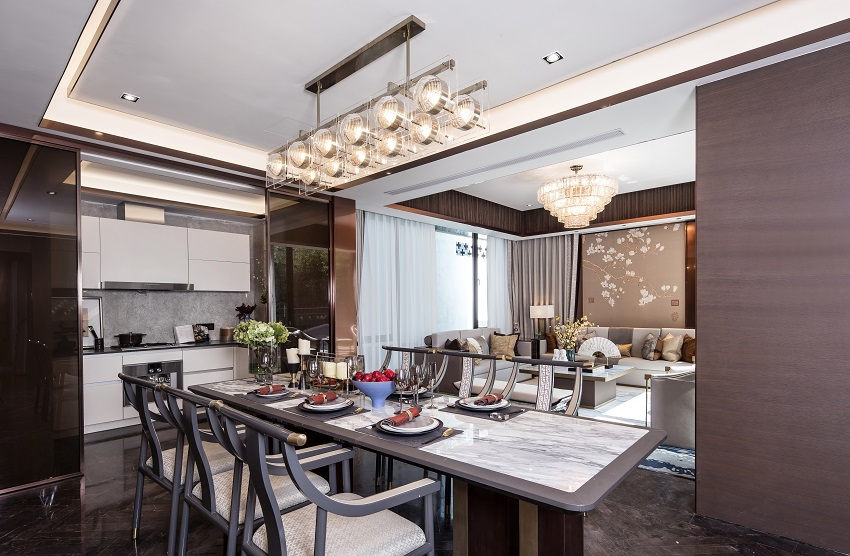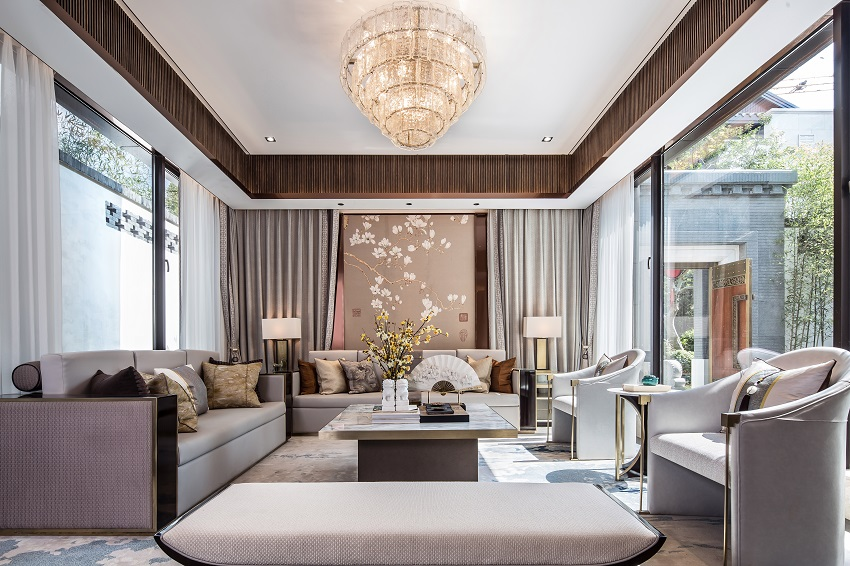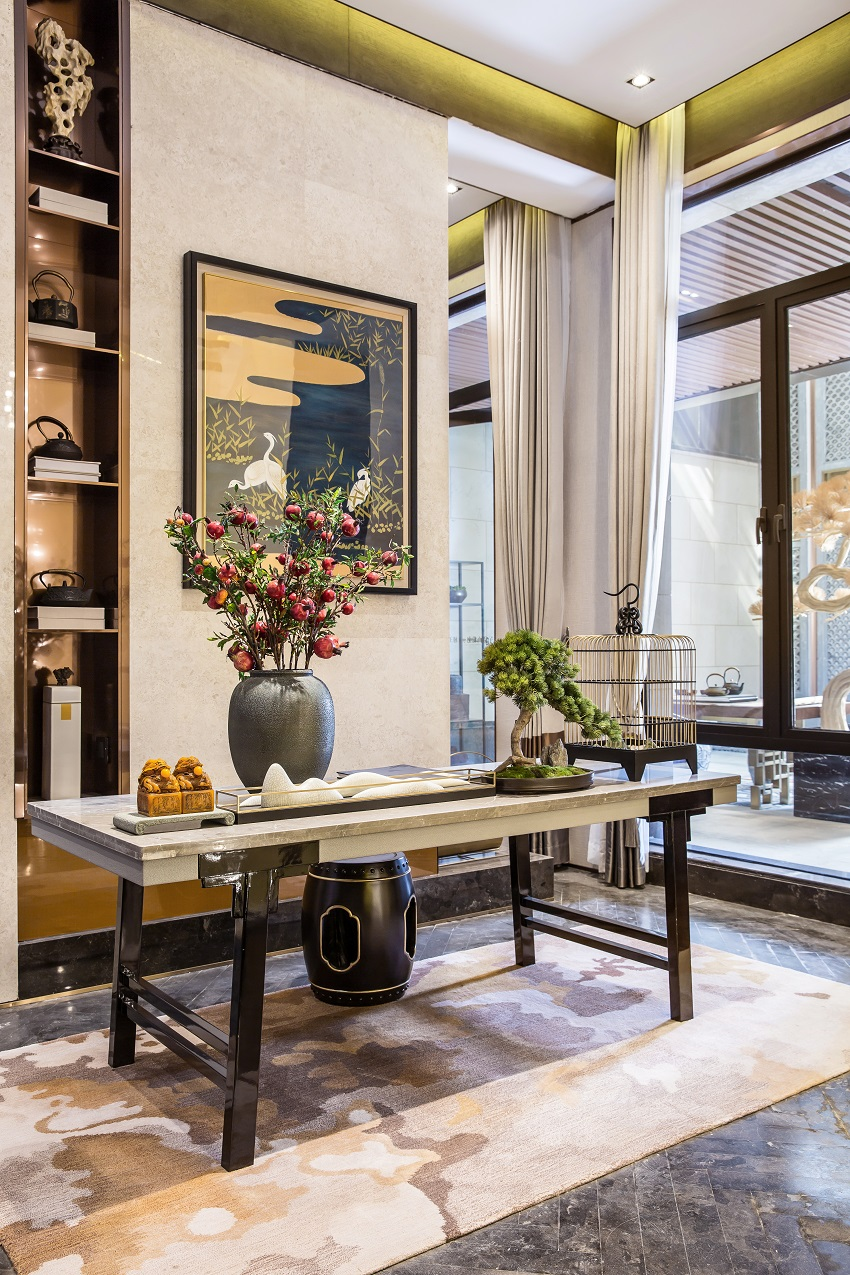▲以上：泽钦室内设计作品 泰禾佛山院子

【搜狐焦点家居】：近年来中式的合院别墅很受关注，你主持的中国院子、合肥院子、姑苏院子等项目，这些项目的相同点和差异性在哪里？

【颜少清】：它们是一脉相承的，整体还是以传承文化为主，是一种延续的文化。具体到每一个项目的时候我们考虑了它当地的风俗和人文，也追溯了每一个地域的历史，在整体设计方面我们把当地的特色带入到设计里，所以它有各地不同的特色，像中国院子是比较官派的风格，充满气派；合肥院子有比较多的徽派元素；佛山院子和深圳院子传承岭南文化的人文设计理念。

【搜狐焦点家居】：佛山主要的产业之一就是瓷砖，做项目时会把当地使用比较广泛的材料运用进来吗？

【颜少清】：我们做佛山项目时主要考虑他们那边的饮食习惯，还有当地的历史故事，瓷砖这些材料的运用比较少，我们的项目中，有把佛山的金属工艺品优势带入到了门把手等五金系列的设计里。

【搜狐焦点家居】：我们说应该关注当下的生活，如何正确理解返古风潮？

【颜少清】：我们对古代的历史研究，和现代的生活是不相悖的。从古代圣人的道德经，论语等文化作品和理念，可以了解到我们中国人对事物的一些惯性理解。我们希望按中国人对历史文化的兴趣爱好，去做设计。比如说我们都有家族的传统，讲究辈分、组织修族谱，这在一定程度上起到了文化传承和凝聚人心的作用，对我们的现实生活有一定的正面意义。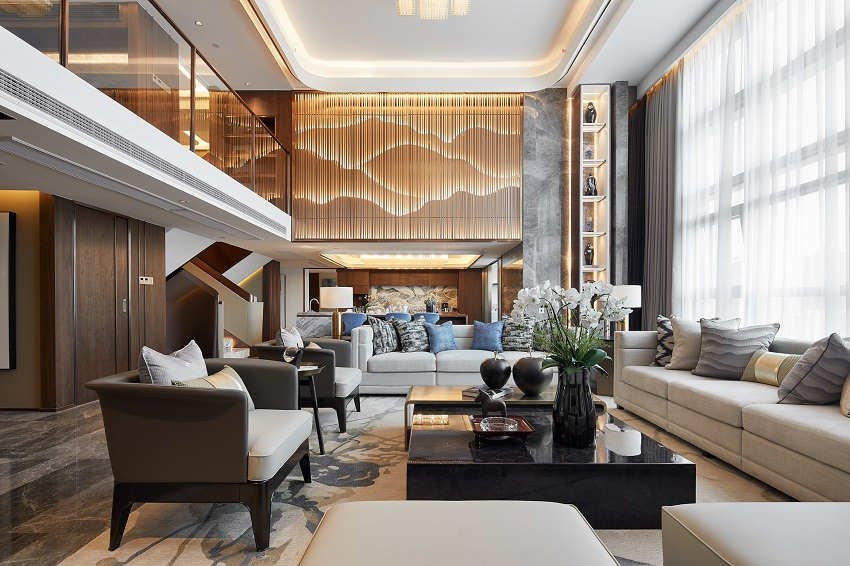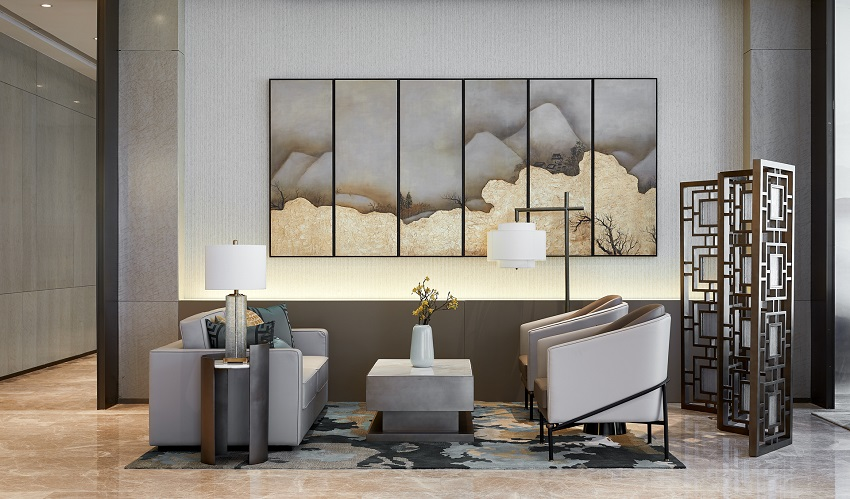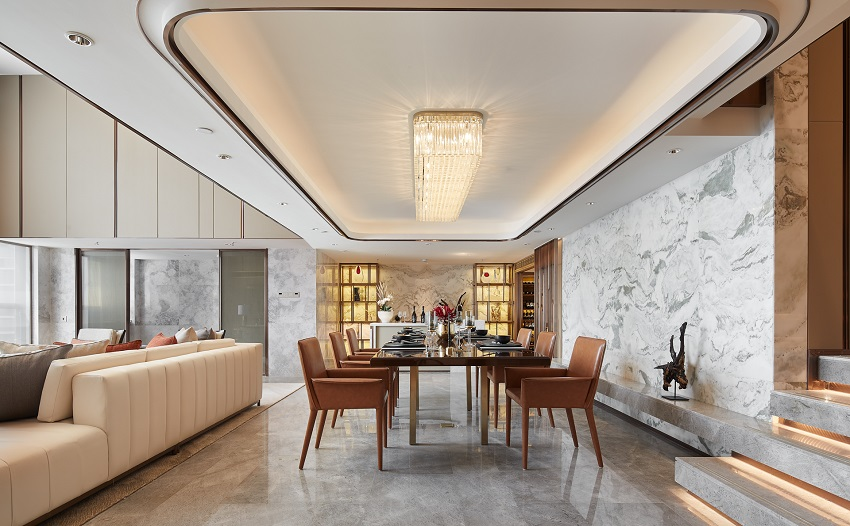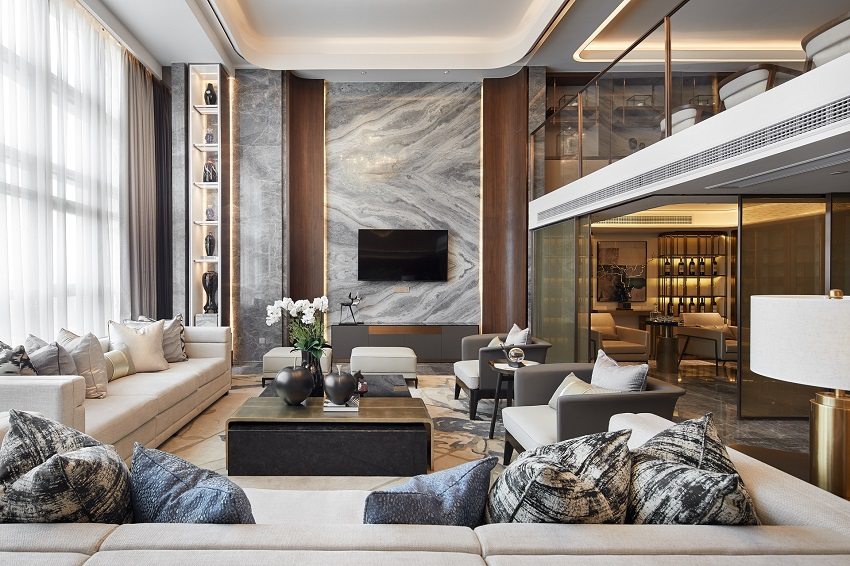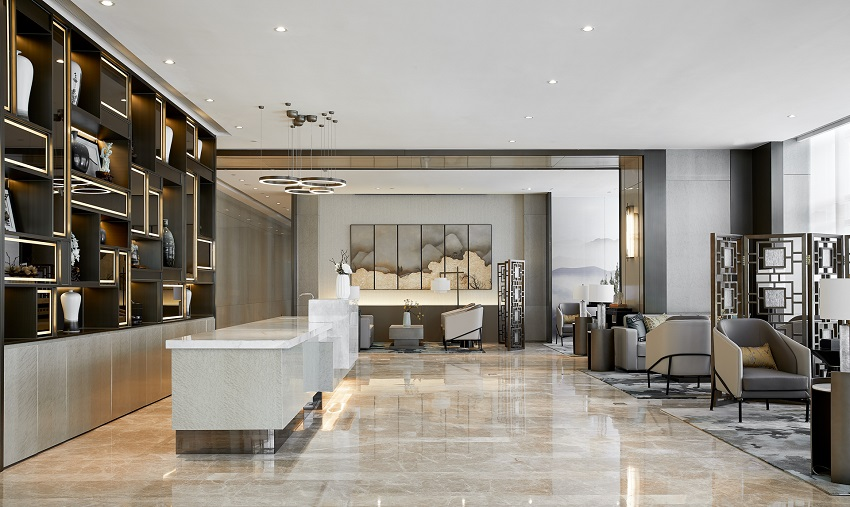▲以上：泽钦室内设计作品 福州泰禾金府天境

【搜狐焦点家居】：讲究辈分、组织修族谱，追溯一定的历史，类似这种行为我可以理解。但是如果业主想要大面积复原古代的建筑，对此你如何理解、以及把这种需求呈现出来的？

【颜少清】：古代建筑是我们精神的传承，我们整体不会以很古老的元素去设计，而是结合设计的标准，框架、空间结构，做一些结构性上的突破，把最美的东西呈现出来。比如说把经典的结构露出来、把这种理念传递出来，而不会完全复制古建筑结构。

【搜狐焦点家居】：中国设计的发展有一个过程，之前风迷欧美，后来照搬香港，你认为现在的中国设计具备了什么样的独特气质吗？

【颜少清】：个人认为现在的中国设计具包容性，变得更加本土化，以更加贴近本土的生活方式，与自然、与环境更好地融合的方向去发展，而不再如以往一味追求奢华。

【搜狐焦点家居】：中式设计如何避免同质化？

【颜少清】：同质化不可避免。因为市场很大，不可控因素又太多。希望更多的设计师能够贴上中式的标签，中式对我来说是一种荣誉，作为中国文化的传播者和实践者。我们先将中式文化，中式建筑“标准化”，再将其扩展出去，这样可以更好地传播中式文化。我希望把中式设计做成越来越靠近中国人的标准，再在局部做一些创新和突破。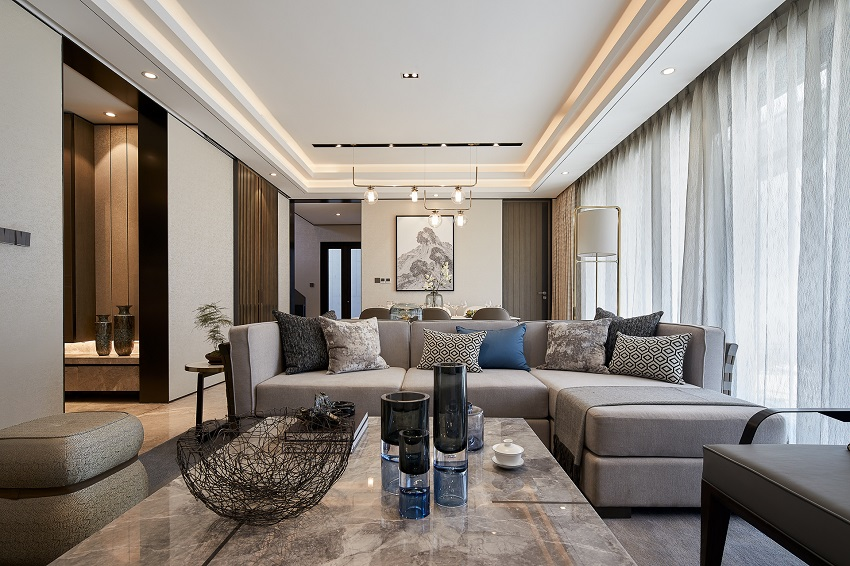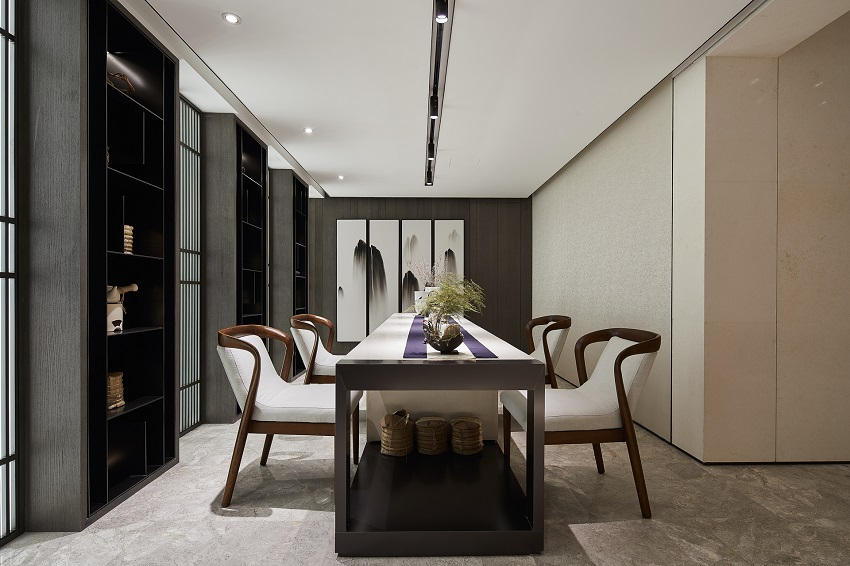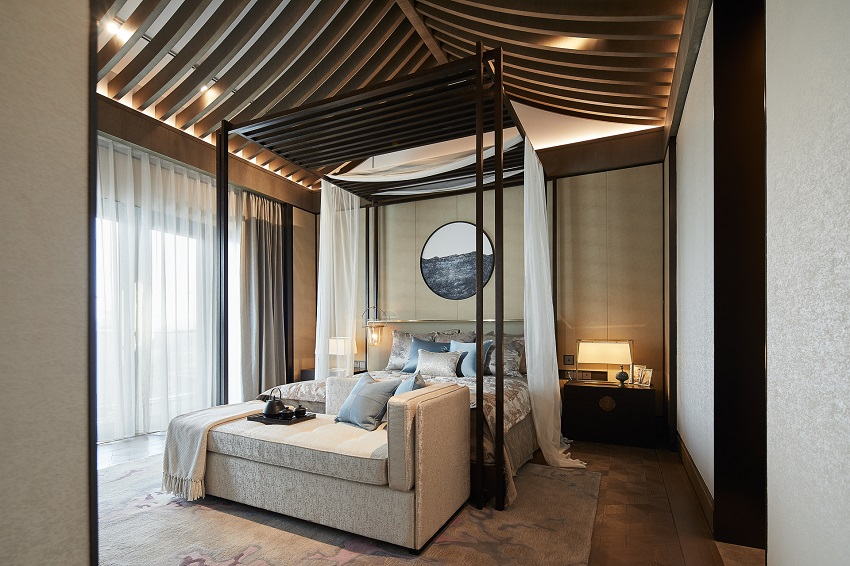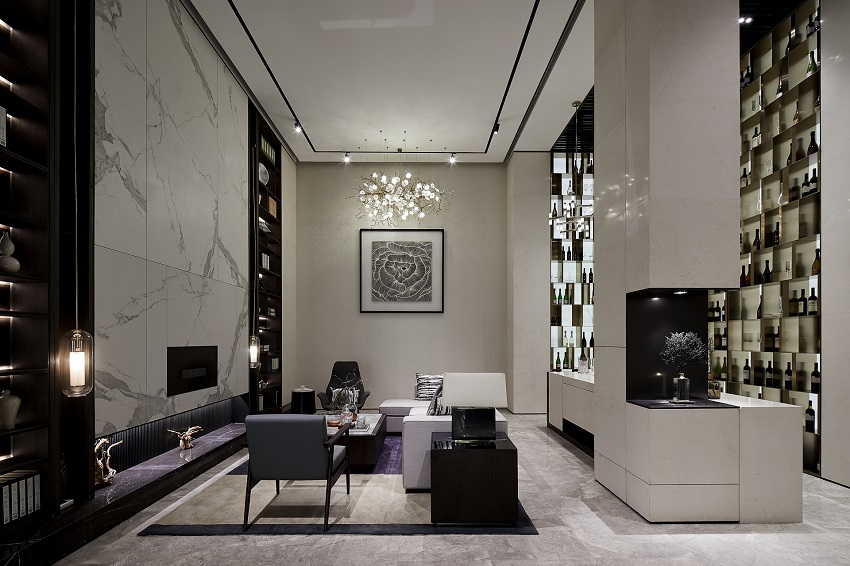▲以上：泽钦室内设计作品 青岛融创红岛壹号院

【搜狐焦点家居】：你主持的中国院子、合肥院子这几个项目都是泰禾出品，泰禾定位“中式文化的践行者”，设计师在实际的项目执行中会不会受到比较大的限制？

【颜少清】：中国文化博大精深且多元化，在整个项目里我们围绕中式来做文章，而每一个地方都有它独特的风俗和人文，我们可以进行很好的发挥。比如对徽派元素的采集和运用，我们曾经去一些古村落做考察和研究，它的一块砖、一块瓦片都可能成为灵感来源。

【搜狐焦点家居】：除了去古村落考察外，灵感创意还来源于其它哪些方式？

【颜少清】：考察当地古村落，研究当地的文化古籍，体验当地的生活，还要和甲方业主沟通，他们其实比我们更了解当地，在沟通过程中很容易碰撞出灵感。

【搜狐焦点家居】：所以说做中式设计和传承，但实际上更多的是依据甲方的需求和文化理解来做中式，而不是真的往远古文化去追寻？

【颜少清】：除了业主的考量，我们还会把诸如书画手法、诗歌意境、玉石文化等带入到空间。比如李白的《望庐山瀑布》，崔颢的《黄鹤楼》，或取其高远淡泊的意境，或借鉴其色彩方面的渲染，还借鉴中国古代的山水画，线描画、人物像等等。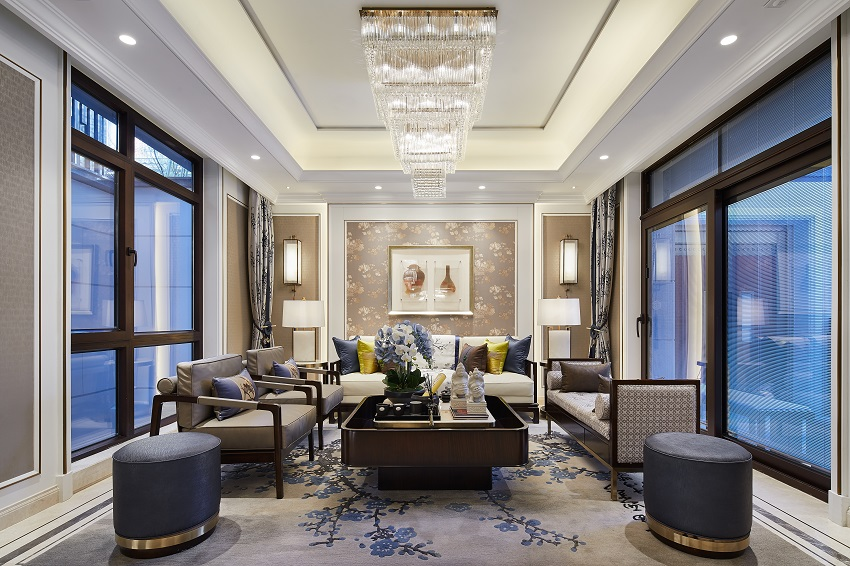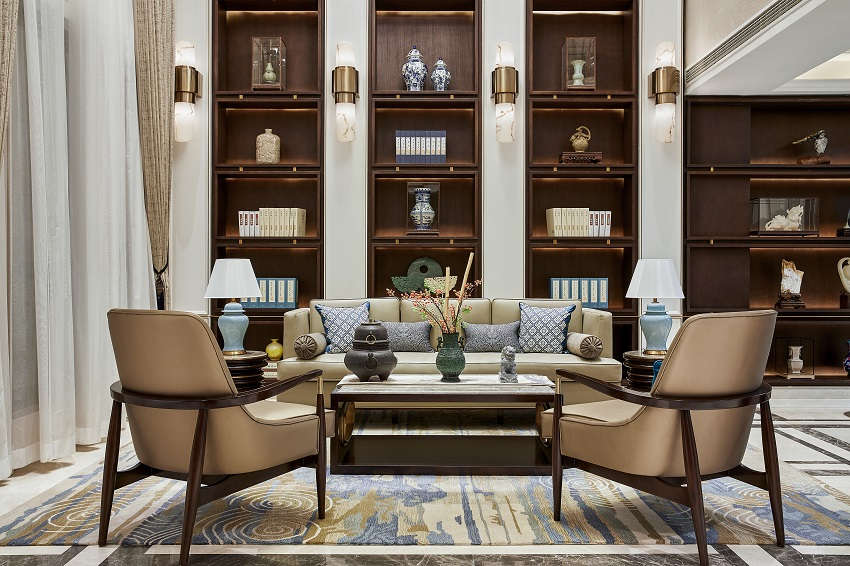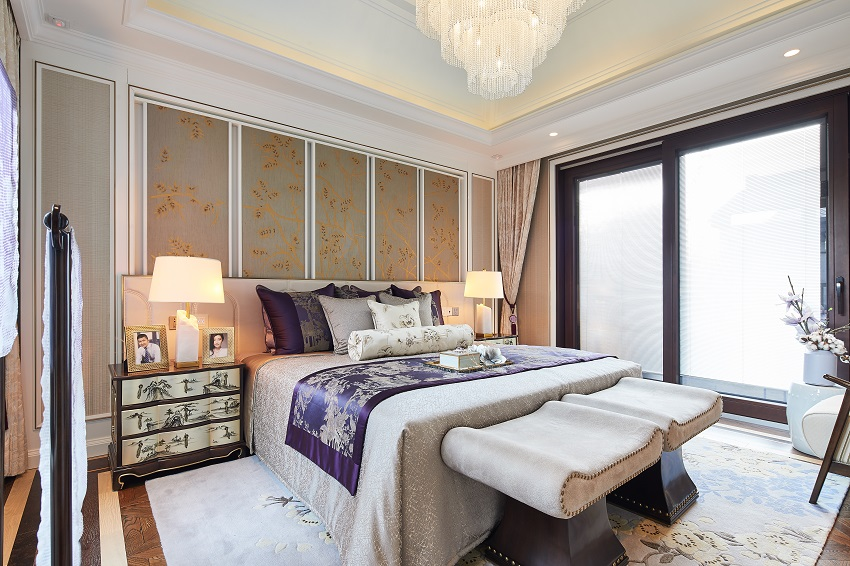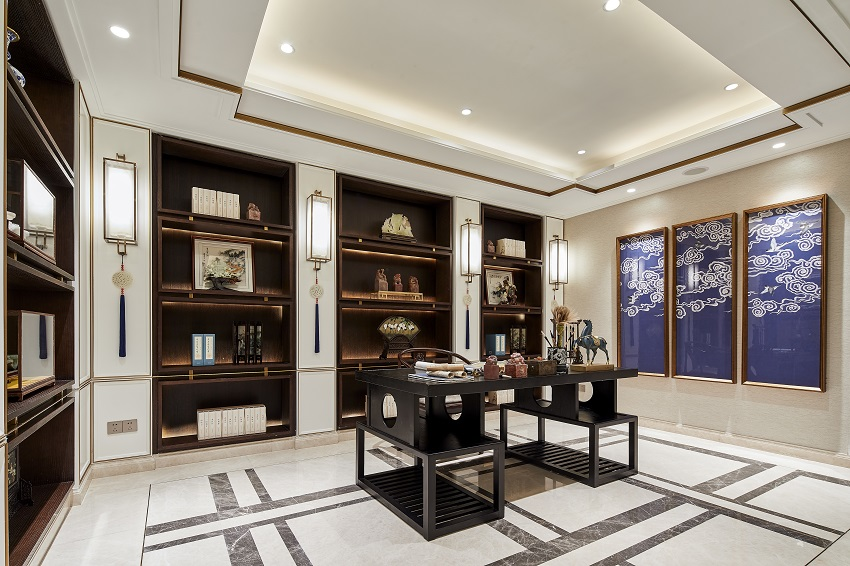▲以上：泽钦室内设计作品：泰禾苏州姑苏院子合院别墅

`声明：本文由入驻焦点开放平台的作者撰写，除焦点官方账号外，观点仅代表作者本人，不代表焦点立场错误信息举报电话： 400-099-0099，邮箱：jubao@vip.sohu.com，或点此进行意见反馈，或点此进行举报投诉。`A B C D E F G H J K L M N P Q R S T W X Y Z
A - B - C - D - E
• A
• 鞍山
• 安庆
• 安阳
• 安顺
• 安康
• 澳门
• B
• 北京
• 保定
• 包头
• 巴彦淖尔
• 本溪
• 蚌埠
• 亳州
• 滨州
• 北海
• 百色
• 巴中
• 毕节
• 保山
• 宝鸡
• 白银
• 巴州
• C
• 承德
• 沧州
• 长治
• 赤峰
• 朝阳
• 长春
• 常州
• 滁州
• 池州
• 长沙
• 常德
• 郴州
• 潮州
• 崇左
• 重庆
• 成都
• 楚雄
• 昌都
• 慈溪
• 常熟
• D
• 大同
• 大连
• 丹东
• 大庆
• 东营
• 德州
• 东莞
• 德阳
• 达州
• 大理
• 德宏
• 定西
• 儋州
• 东平
• E
• 鄂尔多斯
• 鄂州
• 恩施
F - G - H - I - J
• F
• 抚顺
• 阜新
• 阜阳
• 福州
• 抚州
• 佛山
• 防城港
• G
• 赣州
• 广州
• 桂林
• 贵港
• 广元
• 广安
• 贵阳
• 固原
• H
• 邯郸
• 衡水
• 呼和浩特
• 呼伦贝尔
• 葫芦岛
• 哈尔滨
• 黑河
• 淮安
• 杭州
• 湖州
• 合肥
• 淮南
• 淮北
• 黄山
• 菏泽
• 鹤壁
• 黄石
• 黄冈
• 衡阳
• 怀化
• 惠州
• 河源
• 贺州
• 河池
• 海口
• 红河
• 汉中
• 海东
• 怀来
• I
• J
• 晋中
• 锦州
• 吉林
• 鸡西
• 佳木斯
• 嘉兴
• 金华
• 景德镇
• 九江
• 吉安
• 济南
• 济宁
• 焦作
• 荆门
• 荆州
• 江门
• 揭阳
• 金昌
• 酒泉
• 嘉峪关
K - L - M - N - P
• K
• 开封
• 昆明
• 昆山
• L
• 廊坊
• 临汾
• 辽阳
• 连云港
• 丽水
• 六安
• 龙岩
• 莱芜
• 临沂
• 聊城
• 洛阳
• 漯河
• 娄底
• 柳州
• 来宾
• 泸州
• 乐山
• 六盘水
• 丽江
• 临沧
• 拉萨
• 林芝
• 兰州
• 陇南
• M
• 牡丹江
• 马鞍山
• 茂名
• 梅州
• 绵阳
• 眉山
• N
• 南京
• 南通
• 宁波
• 南平
• 宁德
• 南昌
• 南阳
• 南宁
• 内江
• 南充
• P
• 盘锦
• 莆田
• 平顶山
• 濮阳
• 攀枝花
• 普洱
• 平凉
Q - R - S - T - W
• Q
• 秦皇岛
• 齐齐哈尔
• 衢州
• 泉州
• 青岛
• 清远
• 钦州
• 黔南
• 曲靖
• 庆阳
• R
• 日照
• 日喀则
• S
• 石家庄
• 沈阳
• 双鸭山
• 绥化
• 上海
• 苏州
• 宿迁
• 绍兴
• 宿州
• 三明
• 上饶
• 三门峡
• 商丘
• 十堰
• 随州
• 邵阳
• 韶关
• 深圳
• 汕头
• 汕尾
• 三亚
• 三沙
• 遂宁
• 山南
• 商洛
• 石嘴山
• T
• 天津
• 唐山
• 太原
• 通辽
• 铁岭
• 泰州
• 台州
• 铜陵
• 泰安
• 铜仁
• 铜川
• 天水
• 天门
• W
• 乌海
• 乌兰察布
• 无锡
• 温州
• 芜湖
• 潍坊
• 威海
• 武汉
• 梧州
• 渭南
• 武威
• 吴忠
• 乌鲁木齐
X - Y - Z
• X
• 邢台
• 徐州
• 宣城
• 厦门
• 新乡
• 许昌
• 信阳
• 襄阳
• 孝感
• 咸宁
• 湘潭
• 湘西
• 西双版纳
• 西安
• 咸阳
• 西宁
• 仙桃
• 西昌
• Y
• 运城
• 营口
• 盐城
• 扬州
• 鹰潭
• 宜春
• 烟台
• 宜昌
• 岳阳
• 益阳
• 永州
• 阳江
• 云浮
• 玉林
• 宜宾
• 雅安
• 玉溪
• 延安
• 榆林
• 银川
• Z
• 张家口
• 镇江
• 舟山
• 漳州
• 淄博
• 枣庄
• 郑州
• 周口
• 驻马店
• 株洲
• 张家界
• 珠海
• 湛江
• 肇庆
• 中山
• 自贡
• 资阳
• 遵义
• 昭通
• 张掖
• 中卫

1室1厅1厨1卫1阳台

1
2
3
4
5

0
1
2

1

1

0
1
2
3报名成功，资料已提交审核A B C D E F G H J K L M N P Q R S T W X Y Z
A - B - C - D - E
• A
• 鞍山
• 安庆
• 安阳
• 安顺
• 安康
• 澳门
• B
• 北京
• 保定
• 包头
• 巴彦淖尔
• 本溪
• 蚌埠
• 亳州
• 滨州
• 北海
• 百色
• 巴中
• 毕节
• 保山
• 宝鸡
• 白银
• 巴州
• C
• 承德
• 沧州
• 长治
• 赤峰
• 朝阳
• 长春
• 常州
• 滁州
• 池州
• 长沙
• 常德
• 郴州
• 潮州
• 崇左
• 重庆
• 成都
• 楚雄
• 昌都
• 慈溪
• 常熟
• D
• 大同
• 大连
• 丹东
• 大庆
• 东营
• 德州
• 东莞
• 德阳
• 达州
• 大理
• 德宏
• 定西
• 儋州
• 东平
• E
• 鄂尔多斯
• 鄂州
• 恩施
F - G - H - I - J
• F
• 抚顺
• 阜新
• 阜阳
• 福州
• 抚州
• 佛山
• 防城港
• G
• 赣州
• 广州
• 桂林
• 贵港
• 广元
• 广安
• 贵阳
• 固原
• H
• 邯郸
• 衡水
• 呼和浩特
• 呼伦贝尔
• 葫芦岛
• 哈尔滨
• 黑河
• 淮安
• 杭州
• 湖州
• 合肥
• 淮南
• 淮北
• 黄山
• 菏泽
• 鹤壁
• 黄石
• 黄冈
• 衡阳
• 怀化
• 惠州
• 河源
• 贺州
• 河池
• 海口
• 红河
• 汉中
• 海东
• 怀来
• I
• J
• 晋中
• 锦州
• 吉林
• 鸡西
• 佳木斯
• 嘉兴
• 金华
• 景德镇
• 九江
• 吉安
• 济南
• 济宁
• 焦作
• 荆门
• 荆州
• 江门
• 揭阳
• 金昌
• 酒泉
• 嘉峪关
K - L - M - N - P
• K
• 开封
• 昆明
• 昆山
• L
• 廊坊
• 临汾
• 辽阳
• 连云港
• 丽水
• 六安
• 龙岩
• 莱芜
• 临沂
• 聊城
• 洛阳
• 漯河
• 娄底
• 柳州
• 来宾
• 泸州
• 乐山
• 六盘水
• 丽江
• 临沧
• 拉萨
• 林芝
• 兰州
• 陇南
• M
• 牡丹江
• 马鞍山
• 茂名
• 梅州
• 绵阳
• 眉山
• N
• 南京
• 南通
• 宁波
• 南平
• 宁德
• 南昌
• 南阳
• 南宁
• 内江
• 南充
• P
• 盘锦
• 莆田
• 平顶山
• 濮阳
• 攀枝花
• 普洱
• 平凉
Q - R - S - T - W
• Q
• 秦皇岛
• 齐齐哈尔
• 衢州
• 泉州
• 青岛
• 清远
• 钦州
• 黔南
• 曲靖
• 庆阳
• R
• 日照
• 日喀则
• S
• 石家庄
• 沈阳
• 双鸭山
• 绥化
• 上海
• 苏州
• 宿迁
• 绍兴
• 宿州
• 三明
• 上饶
• 三门峡
• 商丘
• 十堰
• 随州
• 邵阳
• 韶关
• 深圳
• 汕头
• 汕尾
• 三亚
• 三沙
• 遂宁
• 山南
• 商洛
• 石嘴山
• T
• 天津
• 唐山
• 太原
• 通辽
• 铁岭
• 泰州
• 台州
• 铜陵
• 泰安
• 铜仁
• 铜川
• 天水
• 天门
• W
• 乌海
• 乌兰察布
• 无锡
• 温州
• 芜湖
• 潍坊
• 威海
• 武汉
• 梧州
• 渭南
• 武威
• 吴忠
• 乌鲁木齐
X - Y - Z
• X
• 邢台
• 徐州
• 宣城
• 厦门
• 新乡
• 许昌
• 信阳
• 襄阳
• 孝感
• 咸宁
• 湘潭
• 湘西
• 西双版纳
• 西安
• 咸阳
• 西宁
• 仙桃
• 西昌
• Y
• 运城
• 营口
• 盐城
• 扬州
• 鹰潭
• 宜春
• 烟台
• 宜昌
• 岳阳
• 益阳
• 永州
• 阳江
• 云浮
• 玉林
• 宜宾
• 雅安
• 玉溪
• 延安
• 榆林
• 银川
• Z
• 张家口
• 镇江
• 舟山
• 漳州
• 淄博
• 枣庄
• 郑州
• 周口
• 驻马店
• 株洲
• 张家界
• 珠海
• 湛江
• 肇庆
• 中山
• 自贡
• 资阳
• 遵义
• 昭通
• 张掖
• 中卫• 手机• 分享
• 设计
免费设计
• 计算器
装修计算器
• 入驻
合作入驻
• 联系
联系我们
• 置顶
返回顶部